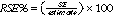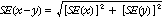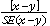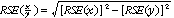4441.0 - Voluntary Work, Australia, 2006
ARCHIVED ISSUE Released at 11:30 AM (CANBERRA TIME) 20/07/2007
 Page tools: .mffeedback,#pjs { display :none; } Enable Javascript to Print PagesPrint All Explanatory Notes Glossary Changes between surveys (Appendix) Data Reliability (Technical Note) Data Source TECHNICAL NOTE DATA RELIABILITY RELIABILITY OF THE ESTIMATES 1 Since the estimates in this publication are based on information obtained from a sample of persons, they are subject to sampling variability. That is, they may differ from those that would have been produced had all persons been included in the survey. One measure of the likely difference is given by the standard error (SE), which indicates the extent to which an estimate might have varied by chance because only a sample of persons was included. There are about two chances in three that the sample estimate will differ by less than one SE from the number that would have been obtained if all persons had been surveyed, and about 19 chances in 20 that the difference will be less than two SEs. 2 Another measure of the likely difference is the relative standard error (RSE), which is obtained by expressing the SE as a percentage of the estimate:3 Space does not allow for the separate indication of the SEs and/or RSEs of all the estimates in this publication. However, RSEs for all these estimates are available free-of-charge on the ABS web site , released in spreadsheet format as an attachment to this publication, Voluntary Work, Australia, 2006 (cat. no. 4441.0). 4 In the tables in this publication, only estimates (numbers, percentages, means and medians) with RSEs less than 25% are considered sufficiently reliable for most purposes. However, estimates with larger RSEs have been included and are preceded by an asterisk (e.g. *3.4) to indicate they are subject to high SEs and should be used with caution. Estimates with RSEs greater than 50% are preceded by a double asterisk (e.g. **2.1) to indicate that they are considered too unreliable for general use. COMPARISON OF ESTIMATES 5 Published estimates may also be used to calculate the difference between two survey estimates. Such an estimate is subject to sampling error. The sampling error of the difference between two estimates depends on their SEs and the relationship (correlation) between them. An approximate SE of the difference between two estimates (x-y) may be calculated by the following formula:6 While the above formula will be exact only for differences between separate and uncorrelated (unrelated) characteristics of subpopulations, it is expected that it will provide a reasonable approximation for all differences likely to be of interest in this publication. SIGNIFICANCE TESTING 7 The statistical significance test for any of the comparisons between estimates was performed to determine whether it is likely that there is a difference between the corresponding population characteristics. The standard error of the difference between two corresponding estimates (x and y) can be calculated using the formula in paragraph 5. This standard error is then used to calculate the following test statistics:8 If the value of this test statistic is greater than 1.96 then we may say there is good evidence of a real difference in the two populations with respect to that characteristic. Otherwise, it cannot be stated with confidence that there is a real difference between the populations. 9 The imprecision due to sampling variability, which is measured by the SE, should not be confused with inaccuracies that may occur because of imperfections in reporting by respondents and recording by interviewers, and errors made in coding and processing data. Inaccuracies of this kind are referred to as non-sampling error, and they occur in any enumeration, whether it be a full count or sample. Every effort is made to reduce non-sampling error to a minimum by careful design or questionnaires, intensive training and supervision of interviewers, and efficient operating procedures. CALCULATING STANDARD ERRORS FOR PROPORTIONS AND PERCENTAGES 10 Proportions and percentages formed from the ratio of two estimates are also subject to sampling errors. The size of the error depends on the accuracy of both the numerator and the denominator. For proportions, where the denominator is an estimate of the number of persons in a group and the numerator is the number of persons in a sub-group of the denominator group, the formula to approximate the RSE is given by:Document Selection These documents will be presented in a new window.